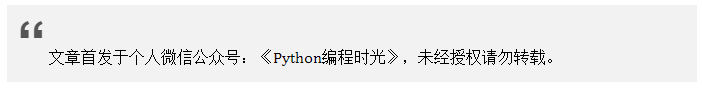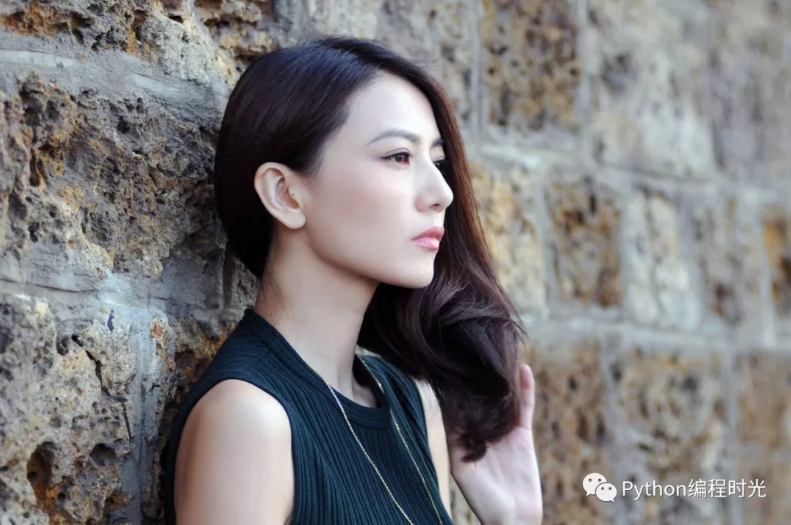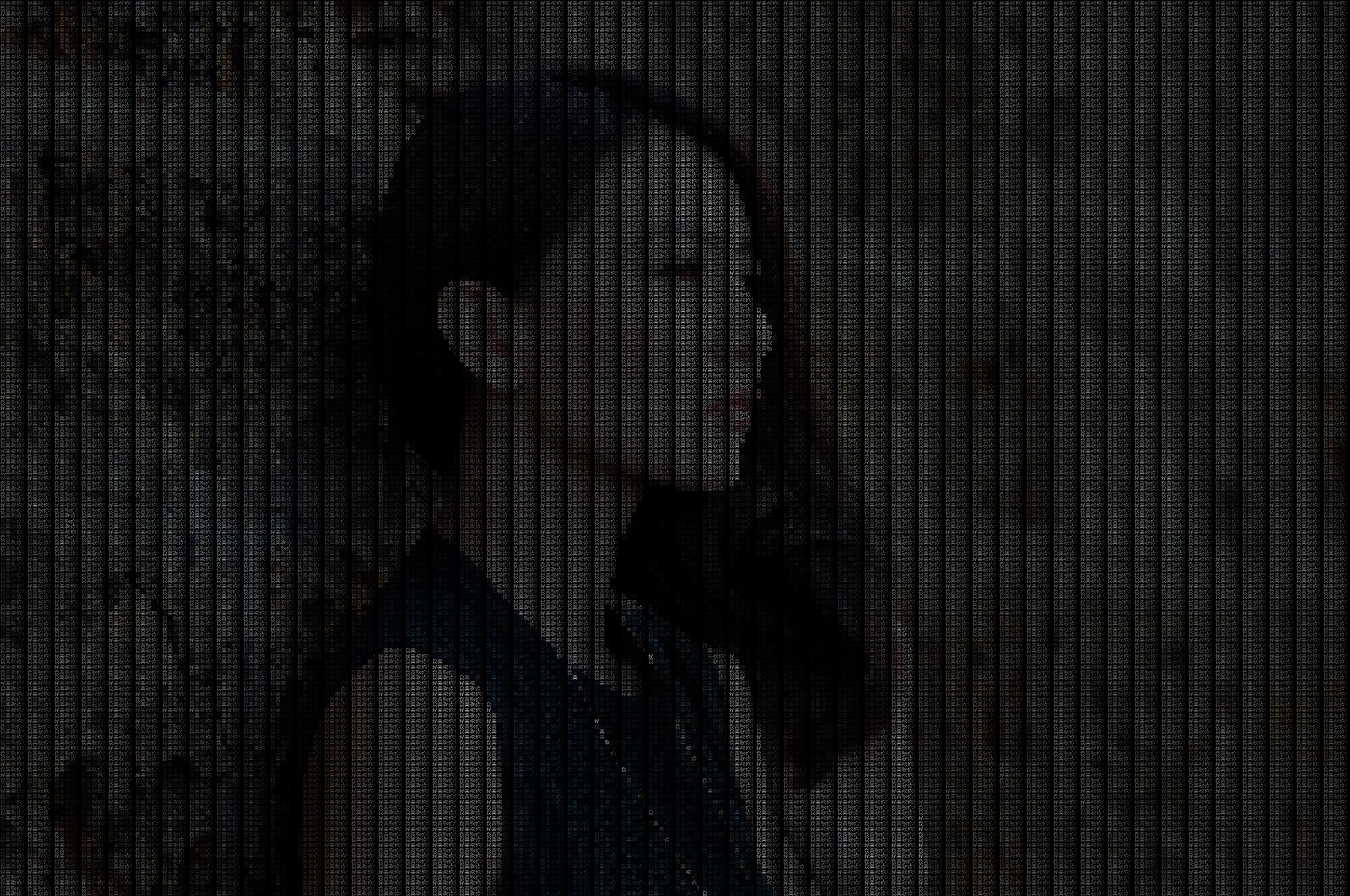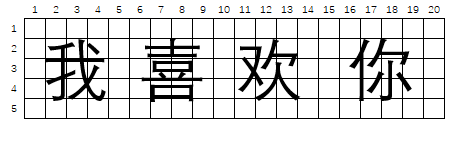# 9.1 情人节来了，教你使用 Python 来表白¶2020年，这个看起来如此浪漫的年份，你还是一个人吗？

2018年的时候，写过一篇介绍如何使用 Python 来表白的文章。imgimg_raw = Image.open(img_path)


img_new = Image.new("RGB", img_raw.size, (0, 0, 0))
draw = ImageDraw.Draw(img_new)
font = ImageFont.truetype('C:/Windows/fonts/Dengl.ttf', font_size)


def character_generator(text):
while True:
for i in range(len(text)):
yield text[i]


for y in range(0, img_raw.size, font_size):
for x in range(0, img_raw.size, font_size):
draw.text((x, y), next(ch_gen), font=font, fill=img_array[x, y], direction=None)


img_new.convert('RGB').save("F://gyy_save.jpeg")


from PIL import Image, ImageDraw, ImageFont

font_size = 7
text = "我喜欢你！"
img_path = "F://gyy.jpeg"

img_raw = Image.open(img_path)
<!--  -->
img_new = Image.new("RGB", img_raw.size, (0, 0, 0))
draw = ImageDraw.Draw(img_new)
font = ImageFont.truetype('C:/Windows/fonts/Dengl.ttf', font_size)

def character_generator(text):
while True:
for i in range(len(text)):
yield text[i]

ch_gen = character_generator(text)

for y in range(0, img_raw.size, font_size):
for x in range(0, img_raw.size, font_size):
draw.text((x, y), next(ch_gen), font=font, fill=img_array[x, y], direction=None)

img_new.convert('RGB').save("F://save.jpeg")